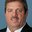Question-and-Answer Resource for the Building Energy Modeling Community
Get s tarted with the Help page

# Part load ratio performance coefficients for chiller and unitary DX systemI am trying to calculate part load ratio performance coefficients for water cooled chiller and unitary system. For Water cooled chiller, EIRfPLR curve coefficients can be get from Energy Plus curve fit tool(excel sheet) in quadratic form? And for unitary DX system, Part load fraction correlation curve was available in Energy Plus - Engineering Reference document (pg no. 818) PLR=0.85+0.15(PLR), can I use this same correlation values for simulation?

edit retag close merge delete

Sort by » oldest newest most votedThe DX coil part load fraction correlation curve PLR=0.85+0.15(PLR) should be used for simulation.The 0.85 in this correlation represents high efficiency DX equipment. Low efficiency DX equipment would use 0.75 to 0.80 (but I have no reference for this).

more

Thank you for your answer. Any idea about EIRfPLR (Energy Input Ratio function of Part load Ratio) curve coefficients for water cooled chiller. Whether that can obtained from Energy Plus curve fit tool excel by using quadratic form. Are any other way to find this coefficients?

Yes the tool can be used for water-cooled chillers. Just input water temps instead of air temps.

1

In order to calculate EIR as a function of PLR, you wouldn't you need to have PLR (Flow Fraction) as the independent variable? And further, to control for temperature on a quadratic curve, wouldn't the temperatures (Evaporator and Condenser Entering Temperatures) have to be held constant for the range of data?

1

The chiller EIRfPLR can't be calculated using the CurveFitTool provided with E+. But it's really only a power fraction curve that roughly goes from 0 at PLR=0 to 1 at PLR=1. I did say "roughly" so bear in mind there is a little bit of a bend to this curve. Plot a chiller curve and you will see.